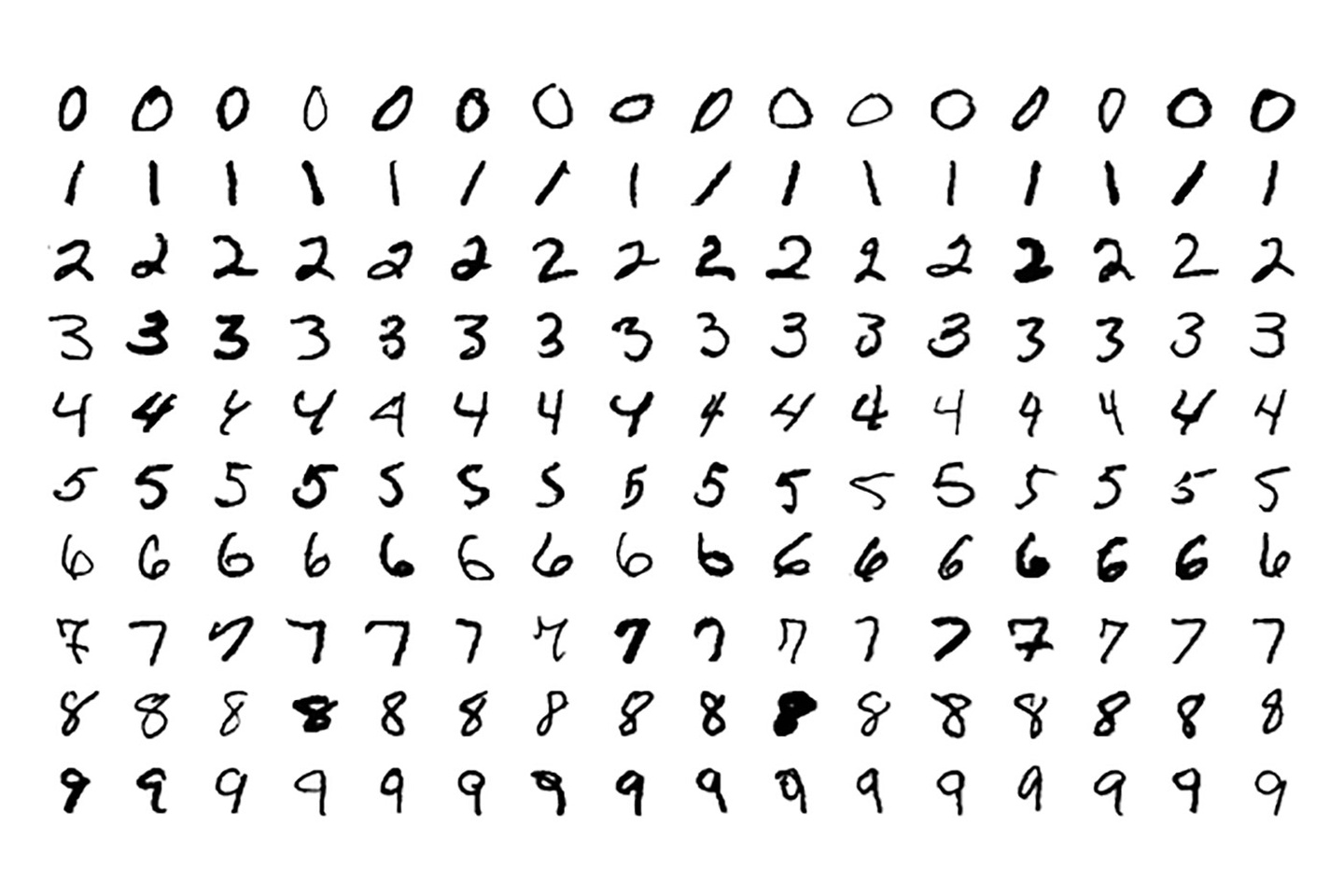TensorFlow是针对大众用户的人工智能（AI）的平台。它是一个拥有大量的社区和强力支持的开源库。TensorFlow提供了一整套用于构建神经网络体系架构的工具，并能训练和发布模型服务。它提供了不同的抽象级别，因此你可以把它用于高级别的剪贴式机器学习过程，或者自己进行更深层的计算的编写。

TensorFlow在tf.layers包中提供了多种类型的层。该模块使得在深度学习模型中创建一个层变得非常容易，且无需考虑许多细节。目前它主要支持用于卷积网络的层的类型。对于其他类型的网络，例如RNN，你可能需要查看tf.contrib.rnntf.nn。最基本的层的类型是全连接层。要实现它，只需要在Dense类中设置输入和大小。其他类型的层可能需要更多的参数，但它们的实现方式可以支持默认行为并节省开发人员的时间。

from tensorflow.examples.tutorials.mnist import input_data

loss = tf.losses.softmax_cross_entropy(labels, output)

correct_prediction = tf.equal(tf.argmax(output, 1), tf.argmax(labels, 1))

accuracy = tf.reduce_mean(tf.cast(correct_prediction, tf.float32))

# Open the session

sess = tf.InteractiveSession()

sess.run(tf.global_variables_initializer())

for i in range(steps):

# Get the next batch

input_batch, labels_batch = next_batch(100)

feed_dict = {x_input: input_batch, y_labels: labels_batch}

# Print the current batch accuracy every 100 steps

if i%100 == 0:

train_accuracy = accuracy.eval(feed_dict=feed_dict)

print(“Step %d, training batch accuracy %g”%(i, train_accuracy))

# Run the optimization step

train_step.run(feed_dict=feed_dict)

# Print the test accuracy once the training is over

print(“Test accuracy: %g”%accuracy.eval(feed_dict={x_input: test_images, y_labels: test_labels}))

input = tf.placeholder(tf.float32, [None, image_size*image_size])

labels = tf.placeholder(tf.float32, [None, labels_size])

TensorFlow的tf.layers包让你只用一行代码就可以完成所有这些工作。你需要提供的就只是输入和层的大小。

output = tf.layers.dense(inputs=input, units=labels_size)

hidden = tf.layers.dense(inputs=input, units=1024, activation=tf.nn.relu)

output = tf.layers.dense(inputs=hidden, units=labels_size)

input2d = tf.reshape(input, [-1,image_size,image_size,1])

conv1 = tf.layers.conv2d(inputs=input2d, filters=32, kernel_size=[5, 5], padding=”same”, activation=tf.nn.relu)

pool1 = tf.layers.max_pooling2d(inputs=conv1, pool_size=[2, 2], strides=2)

pool_flat = tf.reshape(pool1, [-1, 14 * 14 * 32])

hidden = tf.layers.dense(inputs= pool_flat, units=1024, activation=tf.nn.relu)

output = tf.layers.dense(inputs=hidden, units=labels_size)

conv2 = tf.layers.conv2d(inputs=pool1, filters=64, kernel_size=[5, 5], padding=”same”, activation=tf.nn.relu)

pool2 = tf.layers.max_pooling2d(inputs=conv2, pool_size=[2, 2], strides=2)

pool_flat = tf.reshape(pool2, [-1, 7 * 7 * 64])

should_drop = tf.placeholder(tf.bool)

hidden = tf.layers.dense(inputs=pool_flat, units=1024, activation=tf.nn.relu)

dropout = tf.layers.dropout(inputs=hidden, rate=0.5, training=should_drop)

output = tf.layers.dense(inputs=dropout, units=labels_size)Samples from the MNIST test data set (source: Josef Steppan on Wikimedia Commons)